# Sentiment Language Model Trained onAmazon Product Review Data

Generate text in English and analyze sentiment

Released in 2017, this language model uses a single multiplicative LSTM (mLSTM) and byte-level UTF-8 encoding. After training, a "sentiment unit" in the mLSTM hidden state was discovered, whose value directly corresponds to the sentiment of the text.

Number of layers: 27 | Parameter count: 86,245,112 | Trained size: 345 MB |

## Training Set Information

• Amazon Product Review dataset, consisting of 82.83 million unique reviews, from around 20 million users, dating from May 1996-July 2014.

## Performance

• When used for sentiment analysis, fitting a threshold on the sentiment unit achieves

92.3% accuracy on the Large Movie Review Dataset. Using the full 4096-dimensional

hidden state accuracy is increased to 92.88%.

## Examples

### Resource retrieval

Get the pre-trained net:

 In:=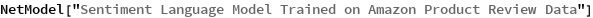Out=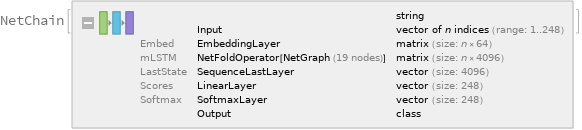### Basic usage

Predict the next character in a piece of text:

 In:=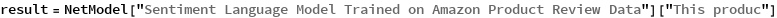Out=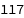The output values correspond to bytes in the UTF-8 encoding (modulo a subtraction by 1). Decode the prediction:

 In:=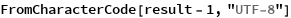Out=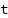Note that since UTF-8 is a variable-length encoding, decoding single byte values may not always make sense:

 In:=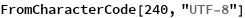Out=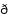### Multiplicative LSTM and UTF-8 encoding

This model features a non-standard multiplicative LSTM (mLSTM), which can be implemented using NetFoldOperator:

 In:=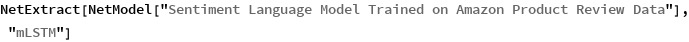Out=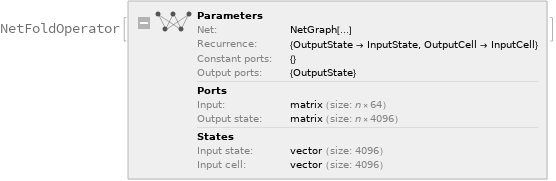Inspect the inner structure of the multiplicative LSTM:

 In:=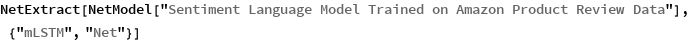Out=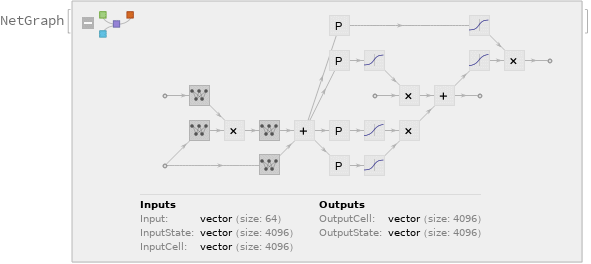This net encodes its input string into a sequence of byte values corresponding to its UTF-8 encoding. Inspect the input encoder:

 In:=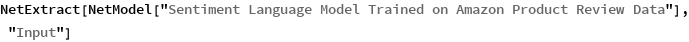Out=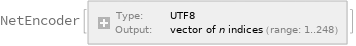The net predicts the next byte of the sequence. UTF-8 allows single byte values in the range 0-247; hence, there are 248 possible outputs. Inspect the input decoder:

 In:=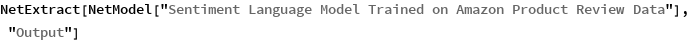Out=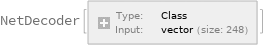### Generation

Write a function to generate text efficiently using NetStateObject. Note that FromCharacterCode[…,"UTF-8"] is used at the end, but output byte values are not guaranteed to produce a valid UTF-8 sequence. In this case, FromCharacterCode will issue messages:

 In:=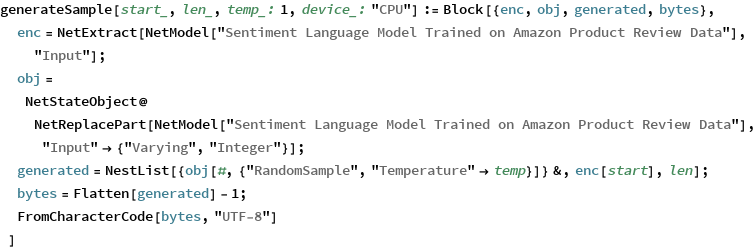Generate for 100 steps using “This produc” as an initial string:

 In:=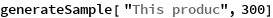Out=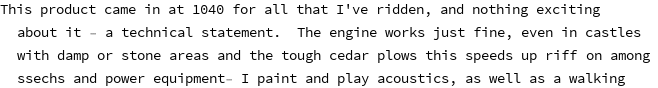The third optional argument is a “temperature” parameter that scales the input to the final softmax. A high temperature flattens the distribution from which characters are sampled, increasing the probability of extracting less likely characters:

 In:=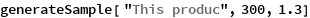Out=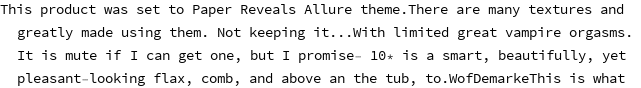Decreasing the temperature sharpens the peaks of the sampling distribution, further decreasing the probability of extracting less likely characters:

 In:=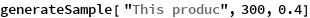Out=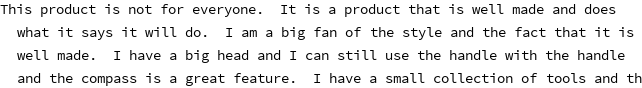Very low temperature settings are equivalent to always picking the character with maximum probability. It is typical for sampling to “get stuck in a loop”:

 In:=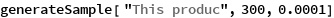Out=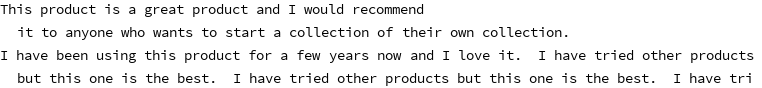Very high temperature settings are equivalent to random sampling. Since the output classes are byte values to be decoded using UTF-8, a very high temperature will almost certainly generate invalid sequences:

 In:=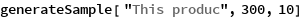Out=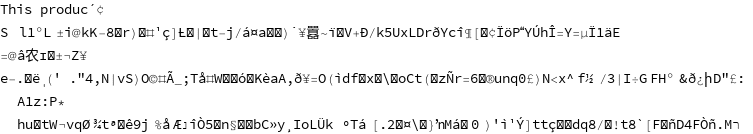### Sentiment analysis

In this model, a single unit of the output state (unit #2389) turns out to directly reflect the sentiment of the text. The final value of this unit can be used as a feature for sentiment analysis. Define a function to extract it:

 In:=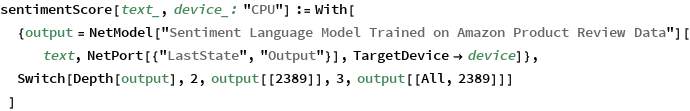Obtain sentiment scores:

 In:=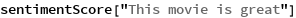Out=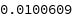In:=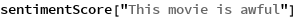Out=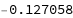Get a subset of the movie review dataset:

 In:=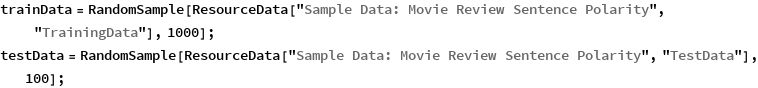In:=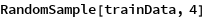Out=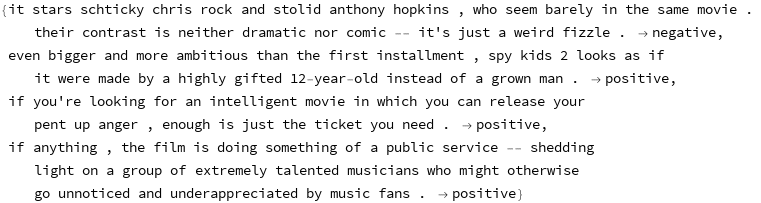Obtain the review scores. If available, GPU evaluation (TargetDevice -> “GPU”) is recommended:

 In:=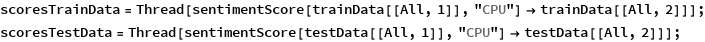Positive and negative reviews are mostly separated by this single score value:

 In:=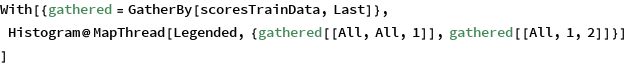Out=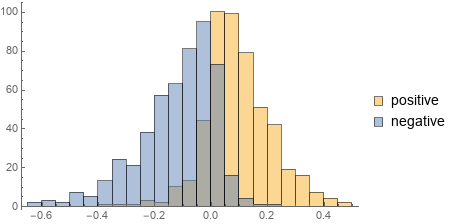Classify the reviews using the score:

 In:=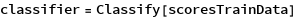Out=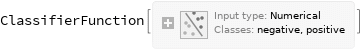Keeping in mind that the training used scalar features from a model not explicitly trained for sentiment analysis, the obtained accuracy is remarkable:

 In:=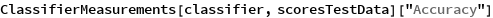Out=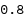### Sentiment visualization

Display the evolution of the sentiment cell as the input is read, with red background corresponding to negative sentiment and green to positive:

 In:=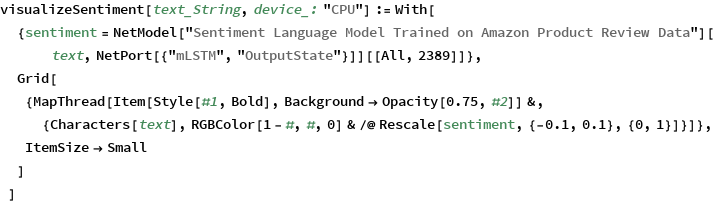As the net reads a positive review, the sentiment cell evolves toward values in the positive score range:

 In:=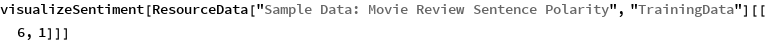Out=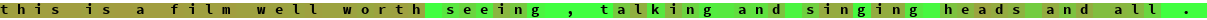In:=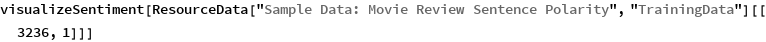Out=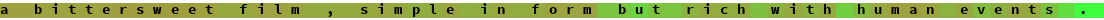As the net reads a negative review, the sentiment cell evolves toward values in the negative score range:

 In:=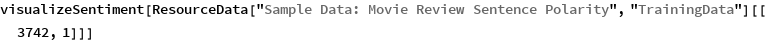Out=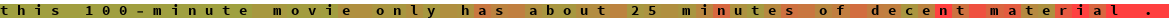In:=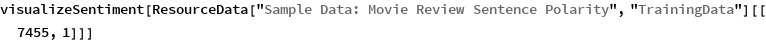Out=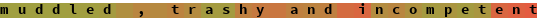### Net information

Inspect the number of parameters of all arrays in the net:

 In:=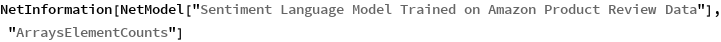Out=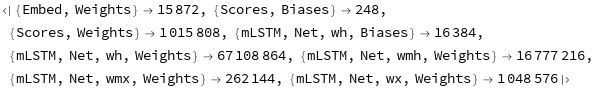Obtain the total number of parameters:

 In:=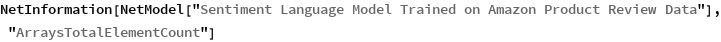Out=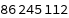Obtain the layer type counts:

 In:=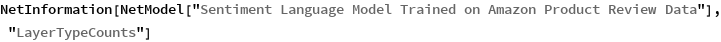Out=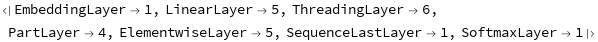### Export to MXNet

Export the net into a format that can be opened in MXNet:

 In:=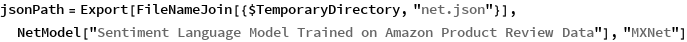Out=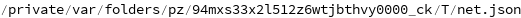Export also creates a net.params file containing parameters:

 In:=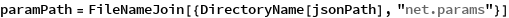Out=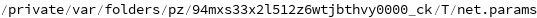Get the size of the parameter file:

 In:=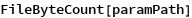Out=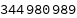The size is similar to the byte count of the resource object:

 In:=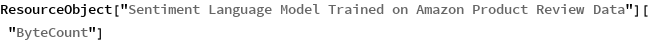Out=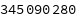## Requirements

Wolfram Language 12.0 (April 2019) or above